# HBSE 6th Class Maths Solutions Chapter 2 Whole Numbers InText Questions

Haryana State Board HBSE 6th Class Maths Solutions Chapter 2 Whole Numbers InText Questions and Answers.

## Haryana Board 6th Class Maths Solutions Chapter 2 Whole Numbers InText Questions

Try These (Page 34)

Question 1.
Write the predecessor and successor of : 19; 1,997; 12,000; 49; 1,00,000; 24,40,701; 1,00,199; 2,08,090.

 Number Predecessor Successor 19 18 20 1,997 1,996 1,998 12,000 11,999 12,001 49 48 50 1,00,000 99,999 1,00,001 24,40,701 24,40,700 24,40,702 1,00,199 1,00,198 1,00,200 2,08,090 2,08,089 2,08,091

Question 2.
Is there any natural number that has no predecessor ?
Yes, 1 has no predecessor.Question 3.
Is there any natural number which has no successor ?
Is there a last natural number ?
No, every natural number has a successor.
No, there is no last natural number.

Try These (Page 35)

Question 1.
Are all natural numbers also whole numbers ?
Yes, all natural numbers are also whole numbers.

Question 2.
Are all whole numbers also natural numbers ?
No, ‘0’ is a whole number but not a natural number.

Question 3.
Which is the smallest whole number ?
‘0’ is the smallest whole number.

Question 4.
Which is the greatest whole number ?
There is no greatest whole number.Try These (Page 37)

Question 1.
Find 4 +5; 2 +6; 3 +5; 1 + 6 using the number line.
(a) 4 + 5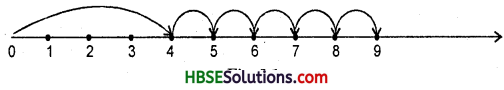The point at the tip of the arrow is 4. Start at 4.
Since, we add 5 to this number so we make 5 jumps to the right; from 4 to 5, 5 to 6, 6 to 7, 7 to 8 and 8 to 9 as shown above in Fig. The tip of the last arrow in the fifth jump is at 9.
∴ The sum of 4 and 5 is 9 i.e. 4 + 5 = 9.

(b) 2 + 6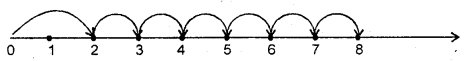The point at the tip of the arrow is 2. Start at 2.
Since we add 6 to this number so we make 6 jumps to the right; from 2 to 3, 3 to 4, 4 to 5, 5 to 6, 6 to 7 and 7 to 8 as shown above in Fig. 2.2. The tip of the last arrow in the sixth jump is at 8.
∴ The sum of 2 and 6 is 8 i.e., 2 + 6 = 8.

(c) 3 + 5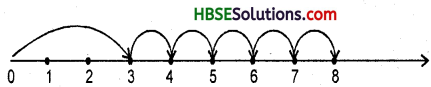The point at the tip of the arrow is 3. Start at 3. Since we add 5 to this number so we make 5 jumps to the right; from 3 to 4, 4 to 5, 5 to 6, 6 to 7 and 7 to 8 as shown above in Fig. 2.3. The tip of the last arrow in the fifth jump is at 8.
∴ The sum of 3 and 5 is 8 i.e., 3 + 5 = 8.

(d) 1 + 6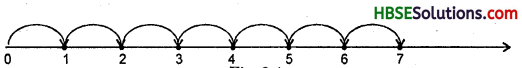The point at the tip of the arrow is 1. Start at 1. Since we add 6 to this number so we make 6 jumps to the right; from 1 to 2, 2 to 3, 3 to 4, 4 to 5, 5 to 6 and 6 to 7 as shown in Fig. 2.4 above. The tip of the last arrow in the sixth jump is at 7.
∴ The sum of 1 and 6 is 7 i.e., 1 + 6 = 7.

Try These (Page 37)

Question 1.
Find 8-3; 6-2; 9-6 using the number line.
(a) 8-3.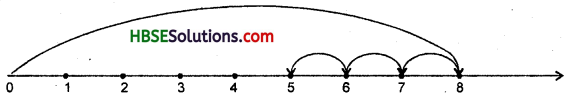The point at the tip of the arrow is 8. Start from 8. Since 3 is being subtracted, so move towards left with 1 jump of 1 unit. Make 3 such jumps. We reach at the point 5.
∴ We get 8 – 3 = 5.(b) 6 – 2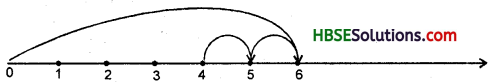The point at the tip of the arrow is 6. Start from 6. Since 2 is being subtracted, so move towards left with 1 jump of 1 unit. Make 2 such jumps. We reach at the point 4.
∴ We get 6-2 = 4.

(c) 9-6.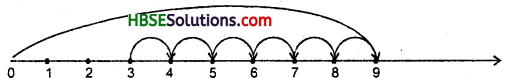The point at the tip of the arrow is 9. Start from 9. Since 6 is being subtracted, so move
towards left with 1 jump of 1 unit. Make 6 such jumps. We reach at the point 3.
∴ We get 9 – 6 = 3.

Try These (Page 37)

Question 1.
Find 2 x 6; 3 x 3; 4 x 2 using number line.
(a) Let us find 2 x 6.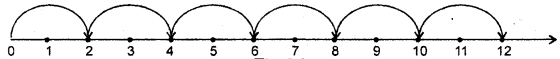Start from ‘0’, move 2 units at a time to the right. Make 6 such moves. We reach at 12. So, we say 2 × 6 = 12.

(b) 3 x 3.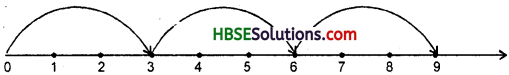Start from ‘0’, move 3 units at a time to the right. Make such 3 moves, we reach at 9. So, we say 3 × 3 = 9.

(c) 4 × 2.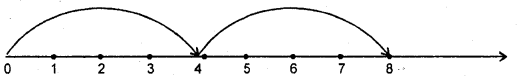Start from ‘0’, move 4 units at a time to the right. Make such 2 moves, we reach at 8. So, we say 4 x 2 = 8.

Try These (Page 45)

Question 1.
(i) 7 + 18 + 13 (ii) 16 + 12 + 4.
Solution:
(i) 7 + 18 + 13 = (7 + 13) + 18
= 20 + 18 = 38
(ii) 16 + 12 + 4 = (16 + 4) + 12
= 20 + 12 = 32.

Question 2.
(i) 25 × 8358 × 4
(ii) 625 × 3759 × 8.
Solution:
(i) 25 × 8358 × 4
= (25 × 4) × 8358
= 100 × 8358
= 835800

(ii) 625 × 3759 × 8
= (625 × 8) × 3759
= 5000 × 3759
= 18795000

Question 3.
(i) (16 ÷ 4) ÷ 2 and 16 ÷ (4 ÷ 2)
(ii) (28 ÷ 14) ÷ 2 and 28 ÷ (14 ÷ 2).
Solution:
(i)( 16 ÷ 4) ÷ 2= 4 ÷ 2 = 2
and 16 ÷ (4 ÷ 2) = 16 ÷ 2 = 8
.-. (16 ÷ 4) ÷ 2 ≠ 16 ÷ (4 ÷ 2)
(ii) (28 + 14) ÷ 2 = 2 ÷ 2 = 1
and 28 ÷ (14 ÷ 2) = 28 ÷ 7 = 4
.-. (28 ÷ 14) ÷ 2 ≠ 28 + (14 ÷ 2)
Hence there is no associative property for division.Try These (Page 48)

Question 1.
(i) 15 × 68
(ii) 17 × 23
(iii) 69 × 78 + 22 × 69.
Solution:
(i) 15 × 68 = 15 × (60 + 8)
= 15 × 60+ 15 × 8 = 900 + 120 = 1020
(ii) 17 × 23 = 17 × (20 + 3)
= 17 × 20 + 17 × 3 = 340 + 51 = 391
(iii) 69 × 78 + 22 × 69
= (78 + 22) × 69 = 100 × 69 = 6900